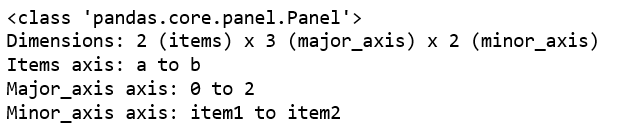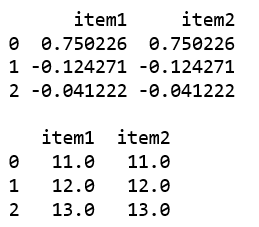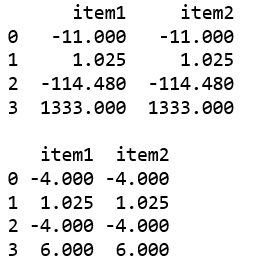Related Articles

# Python | Pandas Panel.clip()

• Last Updated : 01 Jan, 2019

In Pandas, Panel is a very important container for three-dimensional data. The names for the 3 axes are intended to give some semantic meaning to describing operations involving panel data and, in particular, econometric analysis of panel data.

`Panel.clip()` function is used to trim values at input threshold. Thresholds can be singular values or array_like.

Syntax: Panel.clip(lower=None, upper=None, axis=None, inplace=False, *args, **kwargs)

Parameters:Parameters:
lower : Minimum threshold value. All values below this threshold will be set to it.
upper : Maximum threshold value. All values above this threshold will be set to it.
axis : Align object with lower and upper along the given axis.
inplace : Whether to perform the operation in place on the data.

Returns: [Series or DataFrame] Same type as calling object with the values outside the clip boundaries replaced.

Code #1: Creating a Panel using from_dict()

 `# importing pandas module ``import` `pandas as pd ``import` `numpy as np`` ` `df1 ``=` `pd.DataFrame({``'a'``: [``'Geeks'``, ``'For'``, ``'geeks'``], ``                    ``'b'``: np.random.randn(``3``)})``                     ` `data ``=` `{``'item1'``:df1, ``'item2'``:df1}`` ` `# creating Panel ``panel ``=` `pd.Panel.from_dict(data, orient ``=``'minor'``)``print``(panel)`

Output:Code #2: Using clip() function

 `# importing pandas module ``import` `pandas as pd ``import` `numpy as np`` ` `df1 ``=` `pd.DataFrame({``'a'``: [``'Geeks'``, ``'For'``, ``'geeks'``], ``                    ``'b'``: np.random.randn(``3``)})``                     ` `data ``=` `{``'item1'``:df1, ``'item2'``:df1}`` ` `# creating Panel ``panel ``=` `pd.Panel.from_dict(data, orient ``=``'minor'``)`` ` `print``(panel[``'b'``], ``'\n'``)`` ` ` ` `df2 ``=` `pd.DataFrame({``'b'``: [``11``, ``12``, ``13``]})``print``(panel[``'b'``].clip(df2[``'b'``], axis ``=` `0``))`

Output:Code #3: Using clip() function

 `# importing pandas module ``import` `pandas as pd ``import` `numpy as np`` ` `df1 ``=` `pd.DataFrame({``'a'``: [``'Geeks'``, ``'For'``, ``'geeks'``, ``'real'``], ``                    ``'b'``: [``-``11``, ``+``1.025``, ``-``114.48``, ``1333``]})``                     ` `data ``=` `{``'item1'``:df1, ``'item2'``:df1}`` ` `# creating Panel ``panel ``=` `pd.Panel.from_dict(data, orient ``=``'minor'``)`` ` `print``(panel[``'b'``], ``'\n'``)`` ` `print``(panel[``'b'``].clip(``-``4``, ``6``))`

Output:Attention geek! Strengthen your foundations with the Python Programming Foundation Course and learn the basics.

To begin with, your interview preparations Enhance your Data Structures concepts with the Python DS Course. And to begin with your Machine Learning Journey, join the Machine Learning – Basic Level Course

My Personal Notes arrow_drop_up